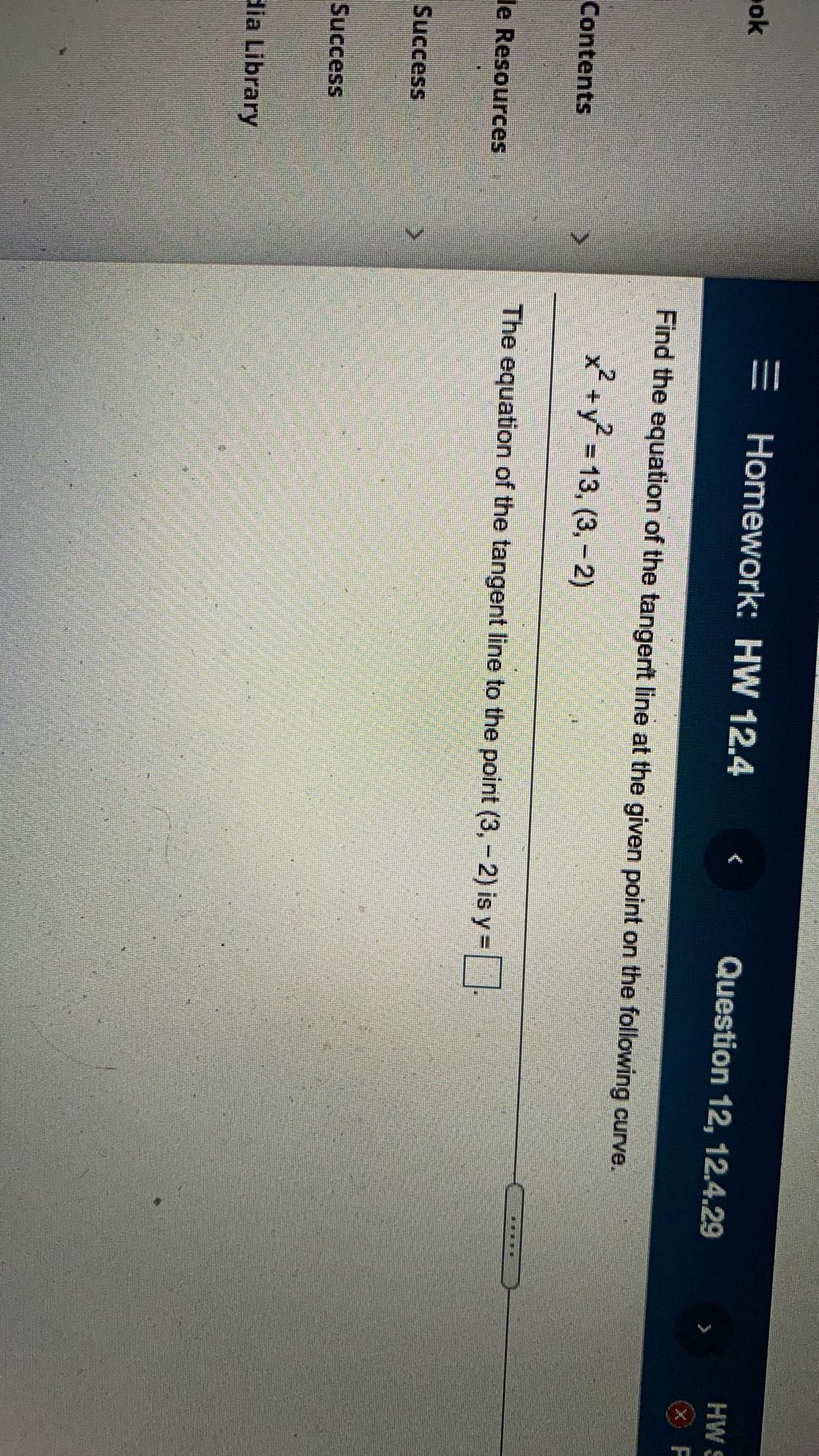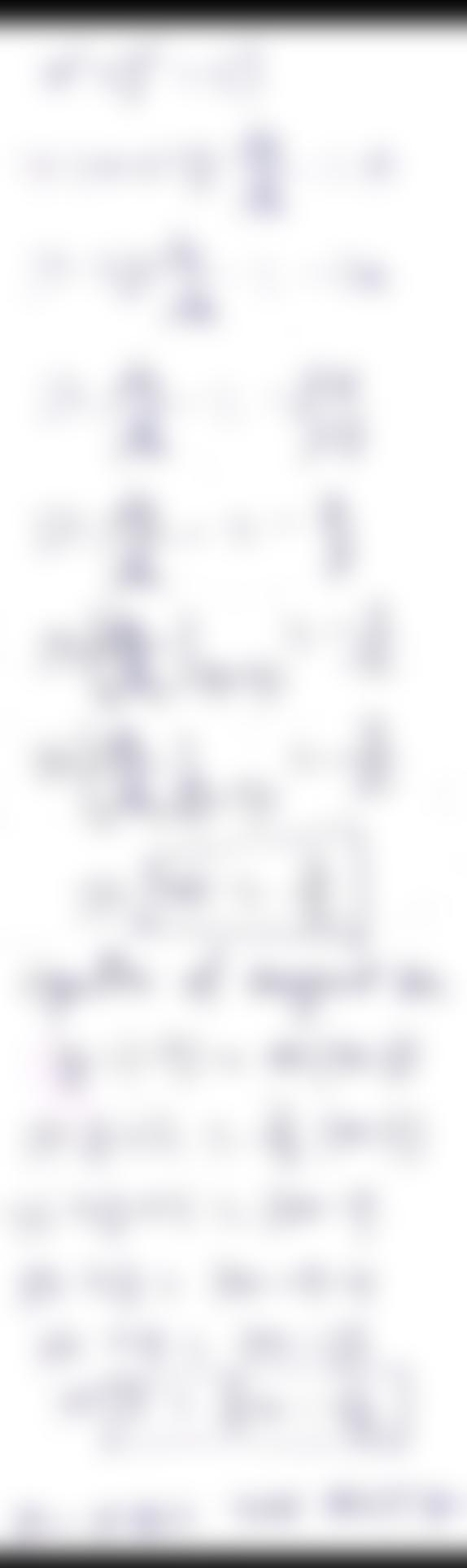Question:

ok = Homework: HW 12.4 Question 12, 12.4.29 HWS Find the equation of the tangent line at the given point on the following curve.ok = Homework: HW 12.4 Question 12, 12.4.29 HWS Find the equation of the tangent line at the given point on the following curve. Contents x? + y2 = 13, (3, - 2) 1 le Resources The equation of the tangent line to the point (3,-2) is y= Success Success dia Library